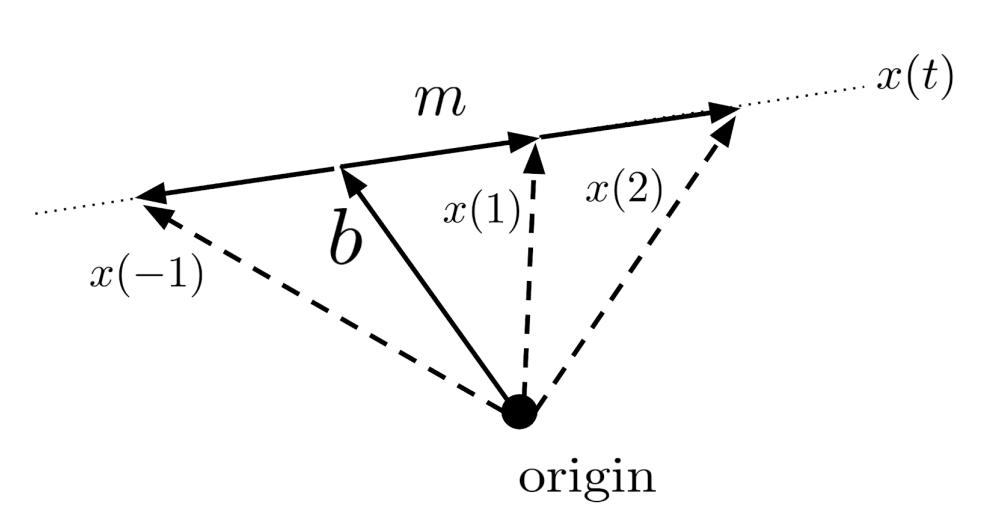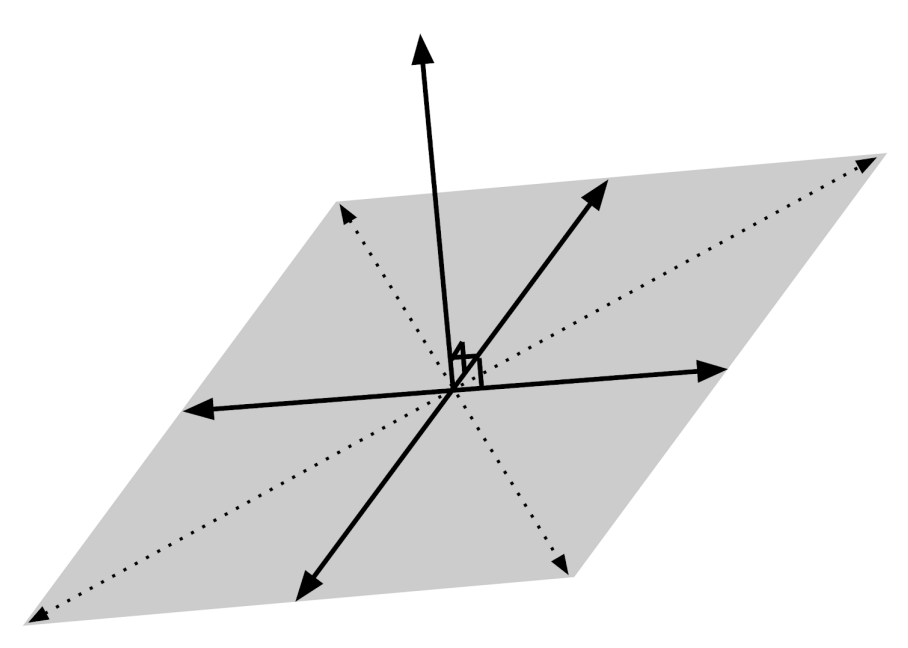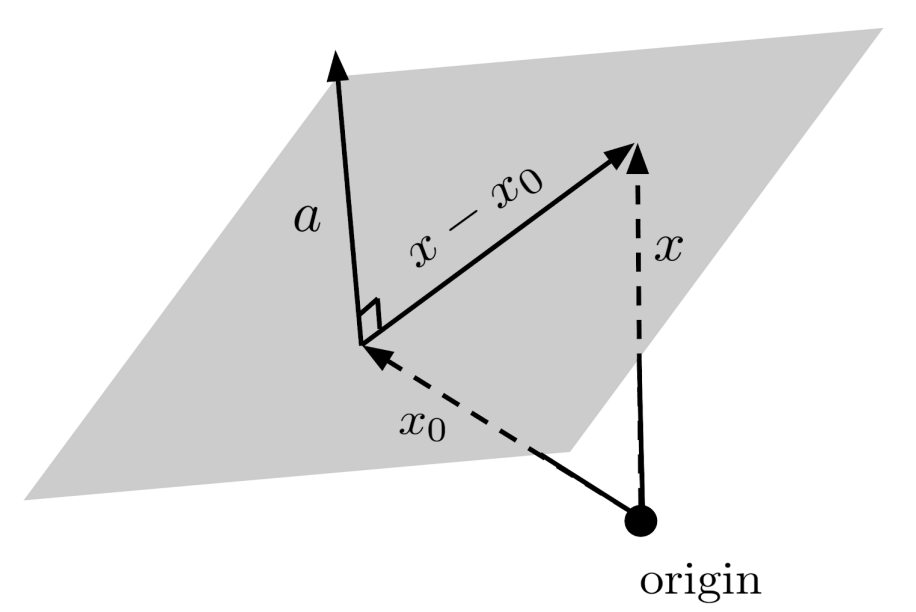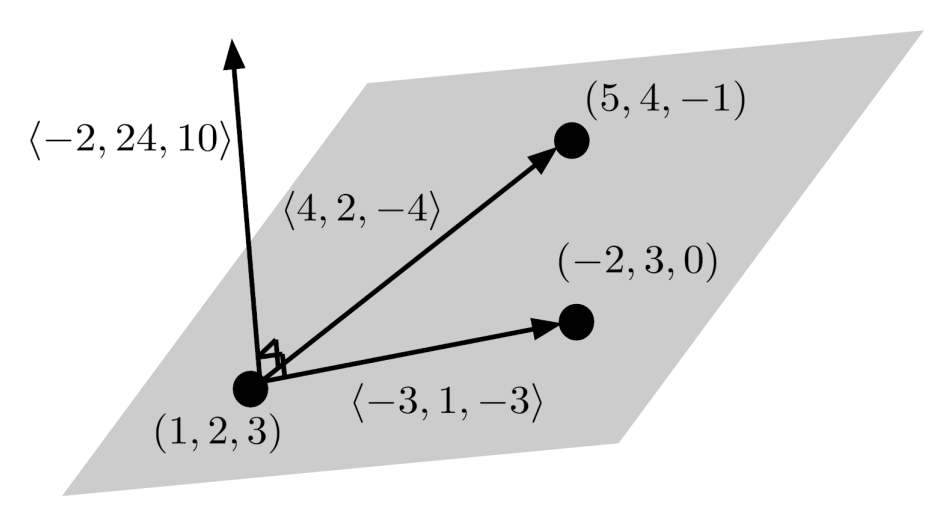# Lines and Planes

This post is part of a series.

A line starts at an initial point and proceeds straight in a constant direction. Thus, we can write the equation of a line as

\begin{align*} x(t)=mt+b \end{align*}

where

• • $b = \left< b_1, b_2, \ldots, b_N \right>$ is the initial point,
• • $m = \left< m_1, m_2, \ldots, m_N \right>$ is the constant direction in which the line travels, and
• • $x(t) = \left< x_1(t), x_2(t), \ldots, x_N(t) \right>$ is the point reached by traveling $t$ units away from $b$ in the direction of $m$.

(Though $x(t)$ is actually a vector, we can also refer to it as the point where the vector lands when the vector is placed at the origin.)## Finding the Equation of a Line

For example, to compute the line between the points $(1,2,3,4)$ and $(5,-2,3,7)$ in 4-dimensional space, we can start by computing the direction $m$ as the difference between the two points:

\begin{align*} m &= \left< 5,-2,3,7 \right> - \left< 1,2,3,4 \right> \\ &= \left< 4,-4,0,3 \right> \end{align*}

Taking $b=\left< 1,2,3,4 \right>$ as our initial point, then, we can express the line as

\begin{align*} x(t) = \left< 4,-4,0,3 \right> t + \left< 1,2,3,4 \right>. \end{align*}

If we wanted to find another point on the line, we could substitute another value for $t$, say, $t=-2$.

\begin{align*} x(2) &= \left< 4,-4,0,3 \right>(-2) + \left< 1,2,3,4 \right> \\ &= \left< -8,8,0,-6 \right> + \left< 1,2,3,4 \right> \\ &= \left< -7,10,3,-2 \right> \end{align*}

## Checking Whether a Point is on a Line

If we wanted to check whether the point $\left< -1,-2,-3,-4 \right>$ is on the line, we could substitute this point for $x(t)$ and try to solve for $t$.

\begin{align*} \left< -1,-2,-3,-4 \right> &= \left< 4,-4,0,3 \right>t + \left< 1,2,3,4 \right> \\ \left< -1,-2,-3,-4 \right> - \left< 1,2,3,4 \right> &= \left< 4,-4,0,3 \right>t \\ \left< -2,-4,-6,-8 \right> &= \left< 4,-4,0,3 \right>t \end{align*}

Setting first components equal, we find $-2=4t$, which implies that $t=-\frac{1}{2}$. But equating second components yields $-4=-4t$, which implies that $t=1$. So, there is no solution that matches all pairs of components, and consequently the point $\left< -1,-2,-3,-4 \right>$ is not on the line.

However, we can verify that the point $\left< 9,-6,3,10 \right>$ is on the line using the same method.

\begin{align*} \left< 9,-6,3,10 \right> &= \left< 4,-4,0,3 \right>t + \left< 1,2,3,4 \right> \\ \left< 9,-6,3,10 \right> - \left< 1,2,3,4 \right> &= \left< 4,-4,0,3 \right>t \\ \left< 8,-8,0,6 \right> &= \left< 4,-4,0,3 \right>t \end{align*}

Equating first components yields $8=4t$ which is valid for $t=2$; equating second components yields $-8=-4t$ which is also valid for $t=2$; equating third components yields $0=0$ which is valid for all choices of $t$; and equating fourth components yields $6=3t$ which is also valid for $t=2$. Thus the point $\left< 9,-6,3,10 \right>$ is on the line because it is simply $x(t)$ evaluated at $t=2$.

## The Equation of a Plane

Now, let’s talk about how to write the equation of a plane in N-dimensional space. A plane can be visualized as a flat sheet that makes a right angle with some particular vector. Thus, a plane just consists of all vectors through some point in the plane, that are perpendicular to a single vector.If $x_0$ is a point in the plane, then the vectors in the plane can be written $x-x_0$, where $x$ represents other points on the plane. These vectors are all perpendicular to a single vector, call it $a$, so their dot product must be zero: $a \cdot (x-x_0) = 0$.Distributing the dot product, we have $a \cdot x - a \cdot x_0 = 0$, and rearranging we have $a \cdot x = a \cdot x_0$. The right-hand side $a \cdot x_0$ is just a constant, so we can simply call it $k$. Thus, we have the general equation for a plane:

\begin{align*} a \cdot x = k \end{align*}

Here, $a$ is a vector that is perpendicular to the plane,$x$ are points on the plane, and $k$ is some constant. Writing $a=\left< a_1, a_2, \ldots, a_N \right>$ and $x = \left< x_1, x_2, \ldots, x_N \right>$, we can expand out the general equation for a plane into an equation consisting only of scalars:

\begin{align*} a_1 x_1 + a_2 x_2 + \ldots + a_N x_N = k \end{align*}

## Finding a Plane Given a Point and Perpendicular Vector

For example, to compute the plane that passes through the point $\left< 5,3,1 \right>$ and has a perpendicular vector of $\left< 1,-1,2 \right>$, we can start by setting up the equation with the perpendicular vector substituted.

\begin{align*} \left< 1,-1,2 \right> \cdot x = k \end{align*}

To solve for $k$, we can simply substitute the point $\left< 5,3,1 \right>$ for $x$ and take the dot product.

\begin{align*} \left< 1,-1,2 \right> \cdot \left< 5,3,1 \right> &= k \\ 1(5) -1(3) +2(1) &= k \\ 4 &= k \end{align*}

Then, we can substitute for $k$ and expand out the dot product in the initial equation.

\begin{align*} \left< 1,-1,2 \right> \cdot x &= 4 \\ \left< 1,-1,2 \right> \cdot \left< x_1, x_2, x_3 \right> &= 4 \\ x_1 - x_2 + 2x_3 &= 4 \end{align*}

Now, suppose we have an equation for a plane, and we want to find the perpendicular vector.

\begin{align*} 2x_1 - 5x_2 + 3 = 10 - x_3 + x_4 \end{align*}

To do this, we can simply organize the equation and convert it to the vector equation of the plane, using the dot product.

\begin{align*} 2x_1 - 5x_2 +3 &= 10 - x_3 + x_4 \\ 2x_1 - 5x_2 + x_3 - x_4 &= 7 \\ \left< 2,-5,1,-1 \right> \cdot \left< x_1, x_2, x_3, x_4 \right> &= 7 \\ \left< 2,-5,1,-1 \right> \cdot x &= 7 \end{align*}

The perpendicular vector is just the first vector in the dot product, $\left< 2,-5,1,-1 \right>$.

## Finding a Plane Given Three Points

Lastly, suppose that we want to find the equation of the plane that contains the three points $(1,2,3)$, $(5,4,-1)$, and $(-2,3,0)$.

To start off, we can find two vectors within the plane by starting at one of the points, say $(1,2,3)$, and computing the displacement vectors to the other two points:

\begin{align*} \left< 5,4,-1 \right> - \left< 1,2,3 \right> &= \left< 4,2,-4 \right> \\ \left< -2, 3, 0 \right> - \left< 1,2,3 \right> &= \left< -3,1,-3 \right> \end{align*}

These displacement vectors are within the plane, so if we can find a vector that is perpendicular to these displacement vectors, then we will have a vector that is normal to the plane.

Since these displacement vectors are 3-dimensional, we can compute their cross product, which yields a perpendicular vector.

\begin{align*} &\left< 4,2,-4 \right> \times \left< -3,1,-3 \right> \\ &= \left< 2(-3)-(-4)(1), -4(-3)-4(-3), 4(1)-2(-3) \right> \\ &= \left< -2, 24, 10 \right> \end{align*}Using this vector as the normal vector of the plane, the equation of the plane becomes

\begin{align*} -2x+24y+10z=k \end{align*}

for some constant $k$. To find the value of $k$, we can simply substitute one of the points in the plane, say, $(1,2,3)$.

\begin{align*} -2(1)+24(2)+10(3)&=k \\ 76 &= k \end{align*}

Thus, the equation of the plane is

\begin{align*} -2x+24y+10z=76 \end{align*}

which can be simplified to

\begin{align*} -x+12y+5z=38. \end{align*}

Looking back, we could have saved some work by using the vector $\left< 2,1,-2 \right>$ instead of the displacement vector $\left< 4,2,-4 \right>$, since $\left< 2,1,-2 \right>$ follows the same direction (it’s just half as long).

The normal vector resulting from the cross product would then have been $\left< -1,12,5 \right>$, which is the normal vector in the fully simplified equation of the plane.

(The vector $\left< -1,12,5 \right>$ points in the same direction as the original normal vector $\left< -2,24,10 \right>$; it’s just half as long.)

## Exercises

Compute the equation of the line that passes through the given points. (You can view the solution by clicking on the problem.)

\begin{align*} 1) \hspace{.5cm} &(1,2,3) \\ &(3,2,1) \end{align*}
Solution:
\begin{align*} & \mbox{answers may vary; one correct answer is} \\ & x(t) = \left< 1,2,3 \right> + \left< 2,0,-2 \right> t \end{align*}

\begin{align*} 2) \hspace{.5cm} &(1,-1,2,-2) \\ &(0,1,4,-3) \end{align*}
Solution:
\begin{align*} & \mbox{answers may vary; one correct answer is} \\ & x(t) = \left< 1,-1,2,-2 \right> + \left< -1,2,2,-1 \right> t \end{align*}

\begin{align*} 3) \hspace{.5cm} &(-5,-1,2,1,3) \\ &(4,4,2,2,0) \end{align*}
Solution:
\begin{align*} & \mbox{answers may vary; one correct answer is} \\ & x(t) = \left< -5,-1,2,1,3 \right> + \left< 9,5,0,1,-3 \right> t \end{align*}

\begin{align*} 4) \hspace{.5cm} &(1,0,-3,-3,0,1) \\ &(3,1,-1,0,2,0) \end{align*}
Solution:
\begin{align*} & \mbox{answers may vary; one correct answer is} \\ & x(t) = \left< 1,0,-3,-3,0,1 \right> + \left< 2,1,2,3,2,-1 \right> t \end{align*}

Check whether the given point $P$ is on the given line. If so, determine the value of $t$ for which $x(t)=P$. (You can view the solution by clicking on the problem.)

\begin{align*} 5) \hspace{.5cm} &P(5,10,5) \\ &x(t)=\left< 1,4,1 \right>+\left< 2,3,2 \right>t \end{align*}
Solution:
\begin{align*} & \mbox{yes; } t=2 \end{align*}

\begin{align*} 6) \hspace{.5cm} &P(2,7,16,0) \\ &x(t)=\left<2,-1,0,3 \right>+\left< 0,1,2,5 \right>t \end{align*}
Solution:
\begin{align*} & \mbox{no} \end{align*}

\begin{align*} 7) \hspace{.5cm} &P(5,6,7,8,9) \\ &x(t)=\left< 1,2,3,4,5 \right>+\left< 5,4,3,2,1 \right>t \end{align*}
Solution:
\begin{align*} & \mbox{no} \end{align*}

\begin{align*} 8) \hspace{.5cm} &P(-2,3,-9,9,-13,12) \\ &x(t)=\left< 3,-2,1,-1,2,-3 \right>+\left< -1,1,-2,2,-3,3 \right>t \end{align*}
Solution:
\begin{align*} & \mbox{yes; } t=5 \end{align*}

Write the equation of the plane that contains the given point $P$ and is perpendicular to the given normal vector $n$. (You can view the solution by clicking on the problem.)

\begin{align*} 9) \hspace{.5cm} &P(0,0,0) \\ &n=\left< 1,2,3 \right> \end{align*}
Solution:
\begin{align*} & x_1 +2x_2 + 3x_3 = 0 \end{align*}

\begin{align*} 10) \hspace{.5cm} &P(1,-1,2) \\ &n=\left< 2,-2,1 \right> \end{align*}
Solution:
\begin{align*} & 2x_1-2x_2+x_3=6 \end{align*}

\begin{align*} 11) \hspace{.5cm} &P(3,0,2,1) \\ &n=\left< 4,-2,0,-5 \right> \end{align*}
Solution:
\begin{align*} &4x_1-x_2-5x_4=7 \end{align*}

\begin{align*} 12) \hspace{.5cm} &P(1,0,1,0,1) \\ &n=\left< 1,-2,-3,2,-1 \right> \end{align*}
Solution:
\begin{align*} & x_1-2x_2-3x_3+2x_4-x_5=-3 \end{align*}

Write the equation of the plane that contains the three given points. (You can view the solution by clicking on the problem.)

\begin{align*} 13) \hspace{.5cm} &(0,0,0)\\ &(0,1,1) \\&(1,0,1) \end{align*}
Solution:
\begin{align*} & x_1+x_2-x_3=0 \end{align*}

\begin{align*} 14) \hspace{.5cm} &(1,3,-5)\\ &(2,-1,2) \\&(1,1,1) \end{align*}
Solution:
\begin{align*} & 5x_1+3x_2+x_3=9 \end{align*}

\begin{align*} 15) \hspace{.5cm} &(2,0,-1)\\ &(-2,-3,-4) \\&(1,2,3) \end{align*}
Solution:
\begin{align*} & 6x_1-19x_2+11x_3=1 \end{align*}

\begin{align*} 16) \hspace{.5cm} &(5,1,1)\\ &(0,3,-2) \\&(0,1,1) \end{align*}
Solution:
\begin{align*} & 3x_2+2x_3=5 \end{align*}

This post is part of a series.

Tags: